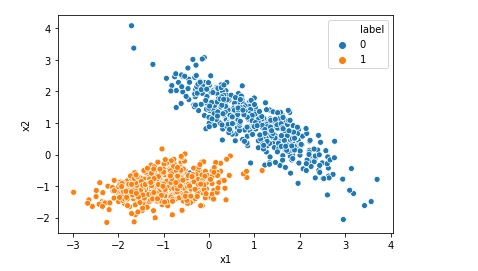# Difference Between Batch, Mini-Batch and Stochastic Gradient Descent

Gradient Descent is one the key algorithm used in Machine Learning. While training machine learning model, we require an algorithm to minimize the value of loss function. Gradient Descent is one of the optimization algorithm , that is used to minimize the loss. There are mainly three types of Gradient Descent algorithm1. Batch Gradient DescentBatch … Continue reading Difference Between Batch, Mini-Batch and Stochastic Gradient Descent# Visualize or Print Random Forest Algorithm Model

As a machine learning engineer you may have created the Random Forest Algorithm Model, but have you ever tried to visualize it. If not , this is the post for you. In this post we will learn how to Visualize or Print Random Forest Algorithm Model in Jupyter notebook. Let us import the required library: … Continue reading Visualize or Print Random Forest Algorithm Model# Random Forest Classification in Python in 10 Lines

Random Forest algorithm is like an ensemble algorithm made of Decision Trees, which comprises more than one decision tree to create a model. It creates more than one tree like conditional control statements to create its model hence it is named as Random Forest. Random Forest machine learning algorithm can be used to solve both … Continue reading Random Forest Classification in Python in 10 Lines# Random Forest Regression in Python in 10 Lines

Random Forest algorithm is like Decision Tree, which comprises more than one decision tree to create a model. Random Forest algorithm is an ensemble method. It creates more than one tree like conditional control statements to create its model hence it is named as Random Forest. Random Forest machine learning algorithm can be used to … Continue reading Random Forest Regression in Python in 10 Lines# Decision Tree Classification in Python in 10 lines

Decision tree machine learning algorithm can be used to solve not only regression but also classification problems. This algorithm creates a tree like conditional control statements to create its model hence it is named as decision tree. In this post we will be implementing a simple decision tree classification model using python and sklearn. First … Continue reading Decision Tree Classification in Python in 10 lines# Print ROC AUC Receiver Operating Characteristic Area Under Curve

The receiver operating characteristic area under curve is a way to measure the performance of a classification model, may be created using algorithms like Logistic Regression. ROC-AUC is basically a graph where we plot true positive rate on y-axis and false positive rate on x-axis. If a model is good the AUC will be close to 1. Area … Continue reading Print ROC AUC Receiver Operating Characteristic Area Under Curve# What is Root Mean Squared Error or RMSE

Root mean squared error or RMSE is a measure of the difference between actual values and predicted values of a machine learning model  like Linear Regression. Root mean squared error is a measure of how well the machine learning model can perform. The lower the RMSE, the better the model. RMSE is always positive, and … Continue reading What is Root Mean Squared Error or RMSE# What is Confusion Matrix in Machine Learning

Not only human beings but also the machine learning models may get confused !! After all Artificial Intelligence mimics a human brain, isn’t it?(pun intended). Imagine yourself as a machine learning engineer and suppose you trained a machine learning classification model successfully today. After the model is trained you checked the accuracy which is 93.0%. Wow … Continue reading What is Confusion Matrix in Machine Learning# Difference between Logistic Regression and Support Vector Machine SVM

Both Logistic Regression and Support Vector Machine or SVM can be used for classification however there is some fundamental difference between them. Lets understand those differences when we are using Support Vector Machine as a classifier. Logistic Regression gives probabilities as output that can be interpreted as confidence in the classification decision however Support Vector … Continue reading Difference between Logistic Regression and Support Vector Machine SVM# Top 5 Assumptions of Linear Regression Algorithm

We can not implement Linear Regression on any given data. If we have got a dataset and we are planning to implement Linear Regression Model on that data we must first check if the given data is inline with the assumptions of Linear Regression. Once the given data agrees to all the assumptions then only … Continue reading Top 5 Assumptions of Linear Regression Algorithm